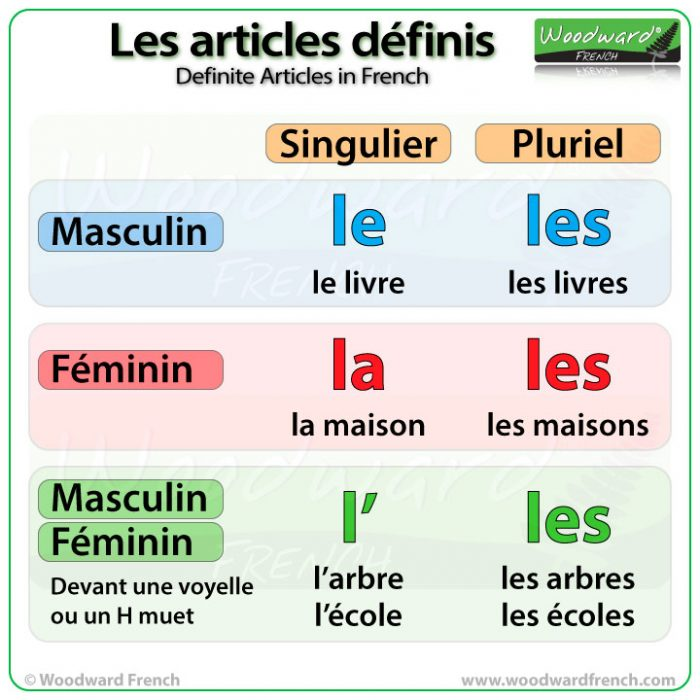Select Page

# Definite Articles in French

### Les articles définis en français(Definite Articles in French)

In English THE is a definite article. – It refers to something specific.

• I need the book. (It is a specific book, one that we both know about)

In English, we are lucky because there is only one form of definite article…. THE

However in French there are different forms of definite article. There is LE, LA, L’, and LES.

So, what is the difference?

The article that is used in French depends on the noun. As we have already seen in a previous lesson, French nouns are either masculine or feminine. They can also come in singular or plural form.

Articles in French need to match:

• the gender (Is the noun masculine or feminine?) and
• the number (Is the noun singular or plural?)

### Definite Articles with Singular Masculine Nouns in French

If a singular noun is masculine then we use either LE, or L’.

The most common masculine definite article (in singular form) is LE.

LE is used before a masculine singular noun that starts with a consonant.

• Le livre (= the book)
• Le fromage (= the cheese)
• Le garçon (= the boy)
• Le père (= the father)

L’ is used before a masculine singular noun that starts with a vowel (= a, e, i, o u) or h (which is silent).

• L’arbre (= the tree)
• L’océan (= the ocean)
• L’homme (= the man)

### Definite Articles with Singular Feminine Nouns in French

If a singular noun is feminine then we use either La or L’.

LA is used before a feminine singular noun that starts with a consonant

• La maison (= the house)
• La porte (= the door)
• La fille (= the girl)
• La voiture (= the car)
• La table (= the table)

L’ is used before a feminine singular noun that starts with a vowel (= a, e, i, o u) or the silent H.

• L’école (= the school)
• L’île (= the island)
• L’université (= the university)
• L’heure (= the hour/the time)

### Definite Articles with Plural Nouns in French

With plural nouns, it is much easier. You always use LES.

It doesn’t matter if the plural noun is masculine or feminine, you use LES before it.

• Le livre — Les livres (= the books)
• Le garçon — Les garçons (= the boys)
• L’arbre — Les arbres (= the trees)
• La maison — Les maisons (= the house)
• La fille — Les filles (= the girls)
• La voiture — Les voitures (= the cars)
• L’école — Les écoles (= the schools)

### French Definite Articles Summary ChartSingulier = Singular
Pluriel = Plural
Masculin = Masculine
Féminin = Feminine
Devant une voyelle ou un H muet = before a vowel or a silent H

Lesson tags: Articles, Grammar, Masculine vs. Feminine
Back to: Basic French Course > French Nouns and Articles